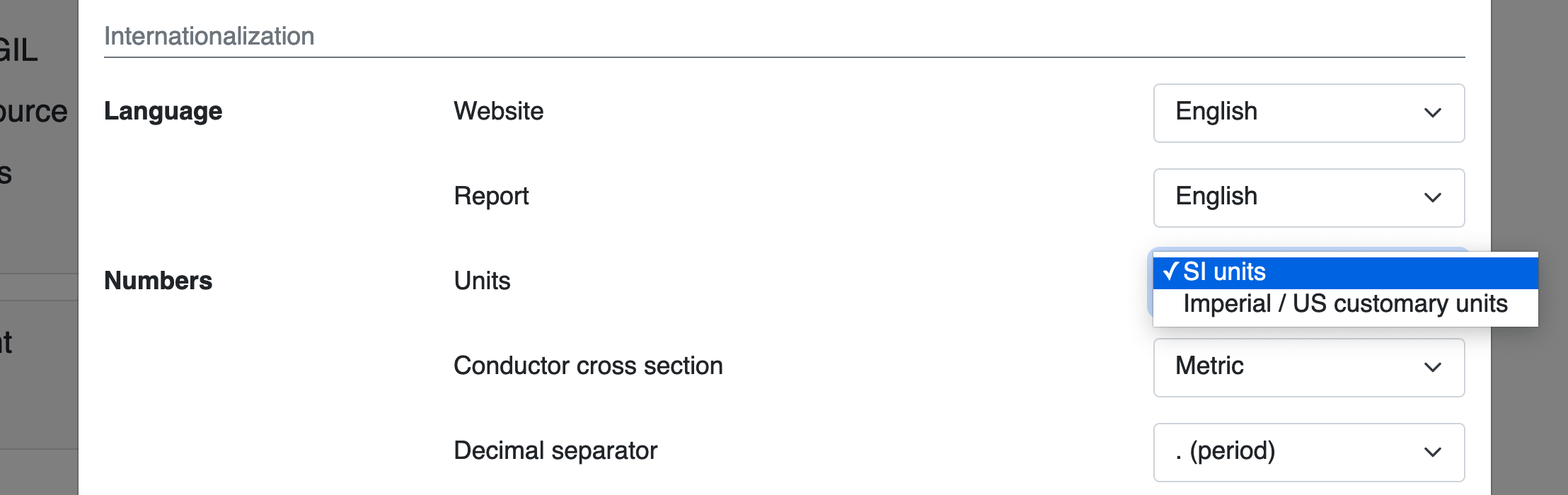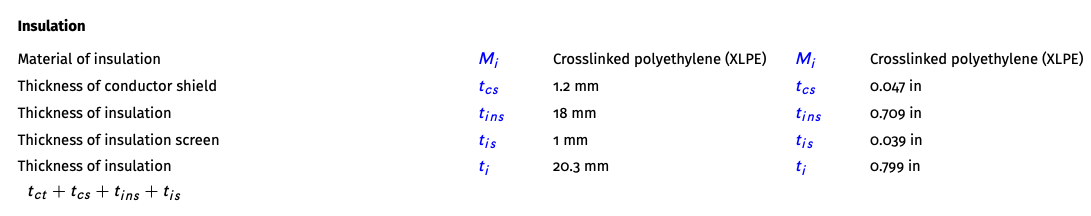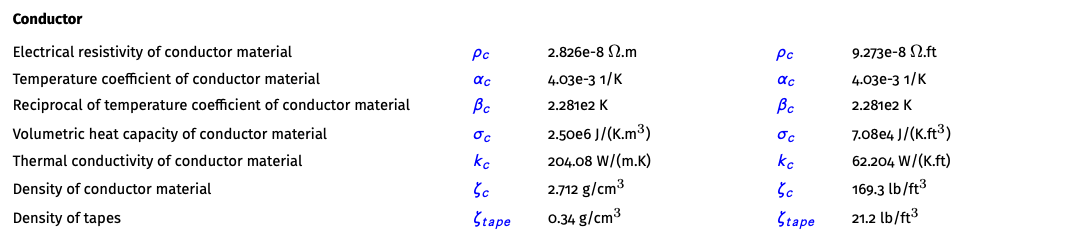# Print reports and datasheets in US customary units

We introduced the possibility to print datasheets and calculation reports optionally in US customary units.

Posted 2022-04-02
Categories: New feature, User guides

Cableizer is based on IEC standards and therefore uses the International System of Units (SI), commonly known as the metric system. With the new version from March 2022 you have now the possibility to create cable datasheets and calculation reports as well in Imperial / US customary units.

### International System of Units

The International System of Units, or short SI, is the international standard for measurement which rests on a foundation of seven defining constants: the cesium hyperfine splitting frequency, the speed of light in vacuum, the Planck constant, the elementary charge (i.e. the charge on a proton), the Boltzmann constant, the Avogadro constant, and the luminous efficacy of a specified monochromatic source. Definitions of all seven SI base units are expressed using an explicit-constant formulation and experimentally realized using a specific practical technique.

Quantity Typical symbol Name Symbol
Time $t$ Second s
Length $l$, $x$, $r$, etc. Meter m
Mass $m$ Kilogram kg
Electric current $I$ Ampere A
Thermodynamic temperature $T$ Kelvin K
Amount of substance $n$ Mole mol
Luminous intensity $I_{V}$ Candela cd

### Settings and reports

In the user profile you choose your prefered unit system: SI units or Imperial / US customary units. This affects only the output of your cable datasheets and calculation reports. In the cable editor and project editors all values have to be entered in SI units and all entered data is kept in SI units in the database and calculation is done exclusively in SI units. For the future, we intend to allow inputs in US units for the cable editor.The reports and the attached cable data sheets will be printed with values according to the selected prefered units system. For cable datasheets, the user has the option to select the other units system.The output in the datasheets and calculation reports is of the same clarity as used to. All values with units as listed in the conversion table below will be converted to the selected units system, actually as data is currently always stored in SI units, the conversion only takes place when printing in Imperial / US customary units.#### Conversions

We use following conversion factors between SI and US units.

Quantity Symbol SI unit US unit Factor Shift
Length L, D, d, r, s, x mm
cm
m
km
in
in
ft
mile
0.0393700787
0.393700787
3.2808399
0.621371192
2
1
0
0
Area A mm$^2$
m$^2$
in$^2$
ft$^2$
0.0015500031
10.7639104
3
–1
Volume V m$^3$ ft$^3$ 35.314666883 –1
Electric current
Reactive Power
I
P
A/km
kvar/m
kvar/km
A/mile
kvar/ft
kvar/mile
1.609344
0.3048
1.609344
0
0
Resistance, Reactance, Impedance R, X, Z $\Omega$/m
$\Omega$/km
$\Omega$/ft
$\Omega$/mile
0.3048
1.609344
1
0
Inductance
Capacitance
Electrical conductance
L
G
H
H/m
F/m
S/m
H/ft
F/ft
S/ft
0.3048
0.3048
0.3048
1
1
1
Losses W W/m W/ft 0.3048 1
Thermal resistivity
Thermal conductivity
Thermal capacitance
T
k
Q
K.m/W
W/(m.K)
J/(m.K)
K.ft/W
W/(K.ft)
J/(ft.K)
3.2808399
0.3048
0.3048
0
1
1
Specific electrical resistivity $\rho$ $\Omega$.m $\Omega$.ft 3.2808399 0
Specific heat capacity cp J/(kg.K) ft$^2$/(s$^2$.K) 10.7639104 –1
Specific volumetric heat capacity $\sigma$ J/(K.m$^3$) J/(K.ft$^3$) 0.028316846 2
Heat transfer coefficient $\alpha$ W/(K.m$^2$) W/(K.ft$^2$) 0.09290304 1
Velocity V cm/s
m/s
in/s
ft/s
0.393700787
3.2808399
1
0
Acceleration g m/s$^2$ ft$^2$ 3.28084 0
Density $\zeta$ g/cm$^3$ lb/ft$^3$ 62.4279606 –2
Mass m kg/km
kg/m
lb/mile
lb/ft
3.54799619
0.3048
1
0
Pressure p hPa
bar
psi
psi
0.0145037738
14.5037738
2
–1
Pulling force F N/mm$^2$ N/in$^2$ 645.16 –3
Kinematic viscosity $\nu$ m$^2$/s cSt 1000000 0
Dynamic viscosity $\eta$ Pa.s cP 1000 0
Stefan Bolzmann constant $\sigma$ W/m$^2$K$^4$ BTU/(hr.ft$^2$.°R$^4$) 0.0302174 3

### From SI derived units

The derived units in the SI are formed by powers, products, or quotients of the base units and are potentially unlimited in number.  Derived units are associated with derived quantities; for example, velocity is a quantity that is derived from the base quantities of time and length, and thus the SI derived unit is metre per second (symbol m/s). The dimensions of derived units can be expressed in terms of the dimensions of the base units.

Combinations of base and derived units may be used to express other derived units. For example, the SI unit of force is the newton (N), the SI unit of pressure is the pascal (Pa) and the pascal can be defined as one newton per square metre (N/m$^2$).

Name Quantity Symbol Unit
Frequency Hertz Hz s$^{-1}$
Force, Weight Newton N kg.m.s$^{-2}$
Pressure Pascal Pa kg.m$^{-1}$.s$^{-2}$
Energy, Work, Heat Joule J kg.m$^2$.s$^{-2}$
Power, Radiant flux Watt W kg.m$^2$.s$^{-3}$
Electric charge Coulomb C s.A
Electrical potential difference (voltage) Volt V kg.m$^2$.s$^{-3}$.A$^{-1}$
Capacitance Farad F kg$^{-1}$.m$^{-2}$.s$^4$.A$^2$
Resistance, Impedance, Reactance Ohm $\Omega$ kg$^{-1}$.m$^{-2}$.s$^3$.A$^2$
Electrical conductance Siemens S
Magnetic flux density Tesla T kg.s$^{-2}$.A$^{-2}$
Inductance Henry H kg.m$^2$.s$^{-2}$.A$^{-2}$
Temperature (relative to 273.15 K) degree Celcius °C K
6
25
12
24
10
9
10
2
9
11
2